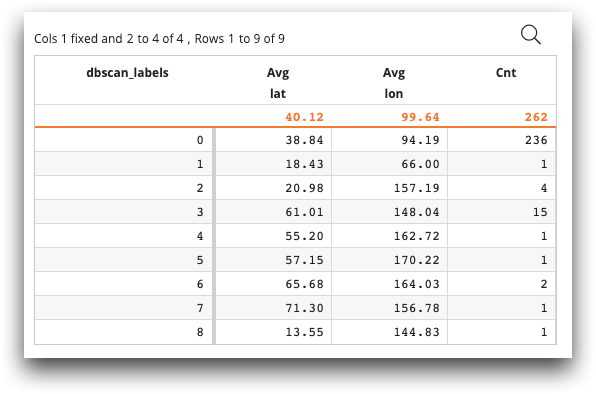# `ten.GetData(ops,table,rows,cols)`

Return a `GetData` object.

## Syntax

``ten.GetData(ops=None,table=None,rows=None,cols=None)``

## Arguments

`ops`
A list representing the set of ops in effect up to the current `<code>`. The default value is None.
`table`
A utf-8 string representing the base path. The default value is None.
`rows`
A list, range, iterator, or NumPy array, with numeric values. The default value is None, which returns all rows.
`cols`
A list of unicode column names (not labels). The default value is None, which returns all columns.

## Methods

`GetData.from_path(table,rows=None,cols=None)`
Modifies a `table` variable of `GetData`.
Arguments:
`table`
A utf-8 string representing the base path.
`rows`
An optional list, range, iterator, or NumPy arrays, with numeric values. The default value is None, which returns all rows.
`cols`
An optional list of unicode column names (not labels). The default value is None.
`GetData.from_ops(ops,rows=None,cols=None)`
Modifies an `ops` variable of `GetData`.
Arguments:
`ops`
A list representing the set of ops.
`rows`
An optional list, range, iterator, or NumPy array, with numeric values (cannot be any empty list). The default value is None, which returns all rows.
`cols`
An optional list of unicode column names (not labels). The default value is None.
`GetData.as_pandas(name_type)`
Returns a pandas `DataFrame` for `name_type` of `'names'` or `'labels'`.
Arguments:
`name_type`
A unicode value, which can be either `'names'` or `'labels'`. The default value is `'names'`.
`GetData.as_arrays()`
Returns a list of NumPy arrays, such as `[np.array([1,2,3],dtype=np.int32),np.array(['a','b','c'])]`.
`GetData.pandas_from_arrays(arrays,names)`
Returns a pandas `DataFrame` for `arrays` (a list of NumPy arrays) and `names` (a list of unicode strings).

This is a helper function to convert arrays to pandas.

Arguments:
`arrays`
A list of NumPy arrays, such as `[np.array([1,2,3],dtype=np.int32),np.array(['a','b','c'])]`.
`names`
A list of unicode strings that will set the names of the columns in the pandas `DataFrame`.

## Returns

A `GetData` object, which contains the variables `ops`, `table`, `rows`, and `cols`.

## Example

The following example retrieves latitude and longitude data from `demos.stations`. The Python code makes use of `DBSCAN`, a density-based clustering algorithm that is imported. The Python code performs the density algorithm to determine the density of the weather stations. `ops`, which now includes the `dbscan_labels` data, now represents the current state of the query. After `ops` is returned, `<tabu>` performs a tabulation of the weather stations, grouped by weather station density. The tabulation includes the average latitude and average longitude at each density level, as well as the count of weather stations at each density level.

```<base table="demos.stations"/>
<code language_="python">
<![CDATA[
from sklearn.cluster import DBSCAN
from ten import GetData as gd
df = gd(ops,table, cols=['lat','lon']).as_pandas()
coords = df.to_numpy()
kms_per_radian = 6371.0088
epsilon = 450 / kms_per_radian
db = DBSCAN(eps=epsilon, min_samples=1, algorithm='ball_tree', metric='haversine').fit(np.radians(coords))
df['dbscan_labels'] = db.labels_
ops = ten.rebase(df)
]]>
</code>
<tabu breaks="dbscan_labels">
<tcol name="avg_lat" source="lat" fun="avg"/>
<tcol name="avg_lon" source="lon" fun="avg"/>
<tcol name="label_cnts" source="dbscan_labels" fun="cnt"/>
</tabu>```